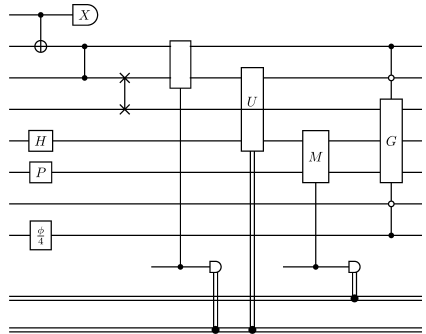# Quantikz.jl

A minimal package for drawing quantum circuits using the quantikz and tikz TeX libraries.

## Examples

using Quantikz
circuit = [
CNOT(1,2), CPHASE(2,3), SWAP(3,4),
H(5), P(6), Id(7),
U("\\frac{\\phi}{4}",8),
Measurement("X",1), Measurement([2,3],2), ClassicalDecision("U",[3,5],2),
Measurement("M",[5,6],1),
MultiControlU("G",[2,8],[7,3],[4,5,6])]## Saving files

Use savecircuit(circuit, filename) for tex, pdf, and png files.

## Custom quantikz TeX code

For each circuit you can get the quantikz table if you want to modify it.

qtable = circuit2table([CNOT(1,2), CPHASE(2,3)])
qtable.qubits, qtable.ancillaries, qtable.bits
(3, 0, 0)
qtable.table
3×4 Matrix{String}:
"\\qw"  "\\ctrl{0}"          "\\qw"        "\\qw"
"\\qw"  "\\targ{}\\vqw{-1}"  "\\ctrl{0}"   "\\qw"
"\\qw"  "\\qw"               "\\ctrl{-1}"  "\\qw"

And after modifications, the table can be turned into a tex string

table2string(qtable)

## Custom Objects

For your CustomQuantumOperation simply define a QuantikzOp(op::CustomQuantumOperation) that converts your object to one of the built-in objects from the examples above.

If you need more freedom for your custom quantum operation, simply define:

• update_table!(table,step,op::CustomQuantumOperation) that directly modifies the quantikz table
• affectedqubits(op::CustomQuantumOperation) that gives the indices of qubits involved in the operation.
• (optional) affectedbits(op) that gives the indices of classical bits in use (empty by default)
• (optional) neededancillaries(op) that gives the number of temporary ancillary qubits to reserve (0 by default)
• (optional) nsteps(op) that gives the number of steps involved in the gate (1 by default)
• (optional) deleteoutputs(op) that gives which qubits to be deleted, e.g., their lines removed (empty by default)

Instead of returning an array of indices affectedqubits can also return the lazy slice ibegin:iend (from EndpointRanges.jl) which tells the layout engine that all qubits are used in this stage of the circuit.